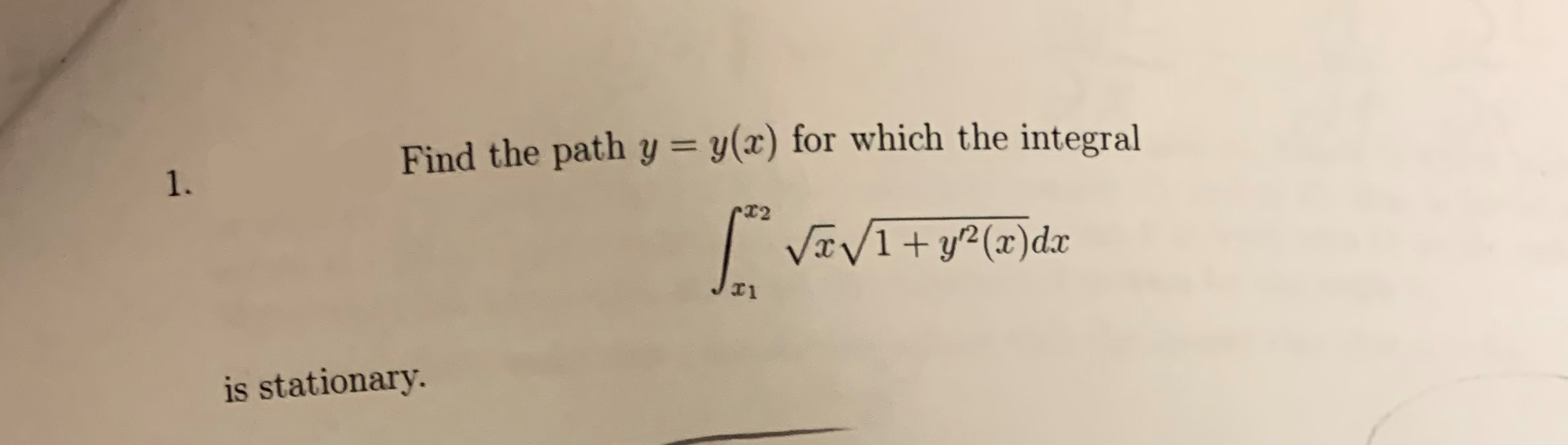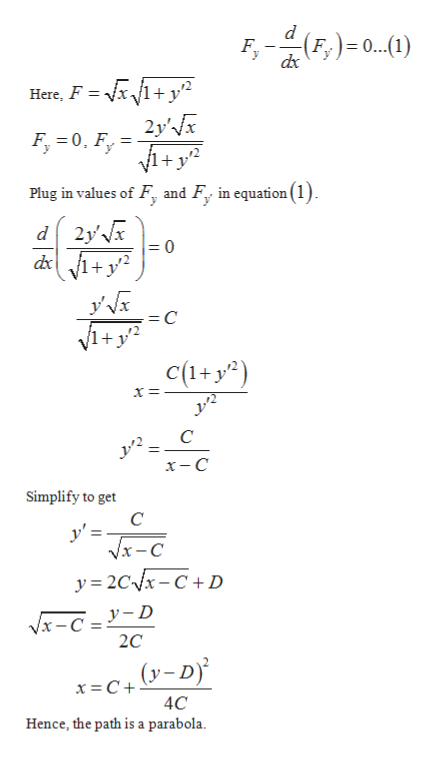# Find the path y = y(x) for which the integral1.r2VaVi+y(xdxis stationary.

Question
37 viewshelp_outlineImage TranscriptioncloseFind the path y = y(x) for which the integral 1. r2 VaVi+y(xdx is stationary. fullscreen
check_circle

Step 1

The path y = y(x) for which the integral is stationa...help_outlineImage Transcriptionclose(F,)=0...-1) Here, F x/1+y2 2y'x i+ y Plug in values of F, and F in equation(1) F 0, F d 2y' = 0 V+y = C 1+ y2 c(+y2) С x-C Simplify to get C y'= Vx-с y 2Cx-C+D x-CIYID 2C y-D (y-D 4C Hence, the path is a parabola fullscreen

### Want to see the full answer?

See Solution

#### Want to see this answer and more?

Solutions are written by subject experts who are available 24/7. Questions are typically answered within 1 hour.*

See Solution
*Response times may vary by subject and question.
Tagged in

### Math